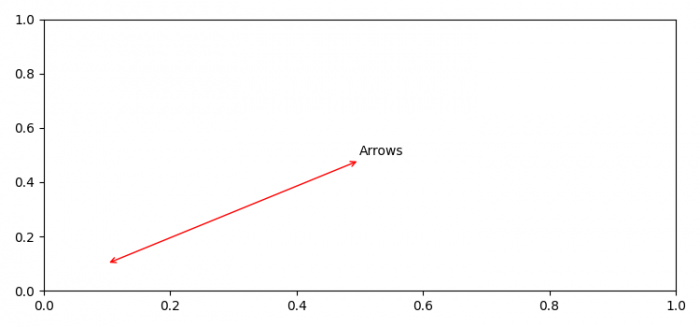# How to make simple double head arrows on the axes in Matplotlib?

To make simple double head arrows on the axes in Matplotlib, we can take the following steps −

• Set the figure size and adjust the padding between and around the subplots.
• Use annotate() method to annotate the point xy with text='Arrows'. Start the tuple and end it for positions. In arrowprops dictionary, use arrowstyle "<->" and color='red'.
• To display the figure, use show() method.

## Example

import matplotlib.pyplot as plt

plt.rcParams["figure.figsize"] = [7.50, 3.50]
plt.rcParams["figure.autolayout"] = True

plt.annotate('Arrows', xy=(0.1, .1), xytext=(0.5, 0.5),
arrowprops=dict(arrowstyle='<->', color='red'))

plt.show()

## Output